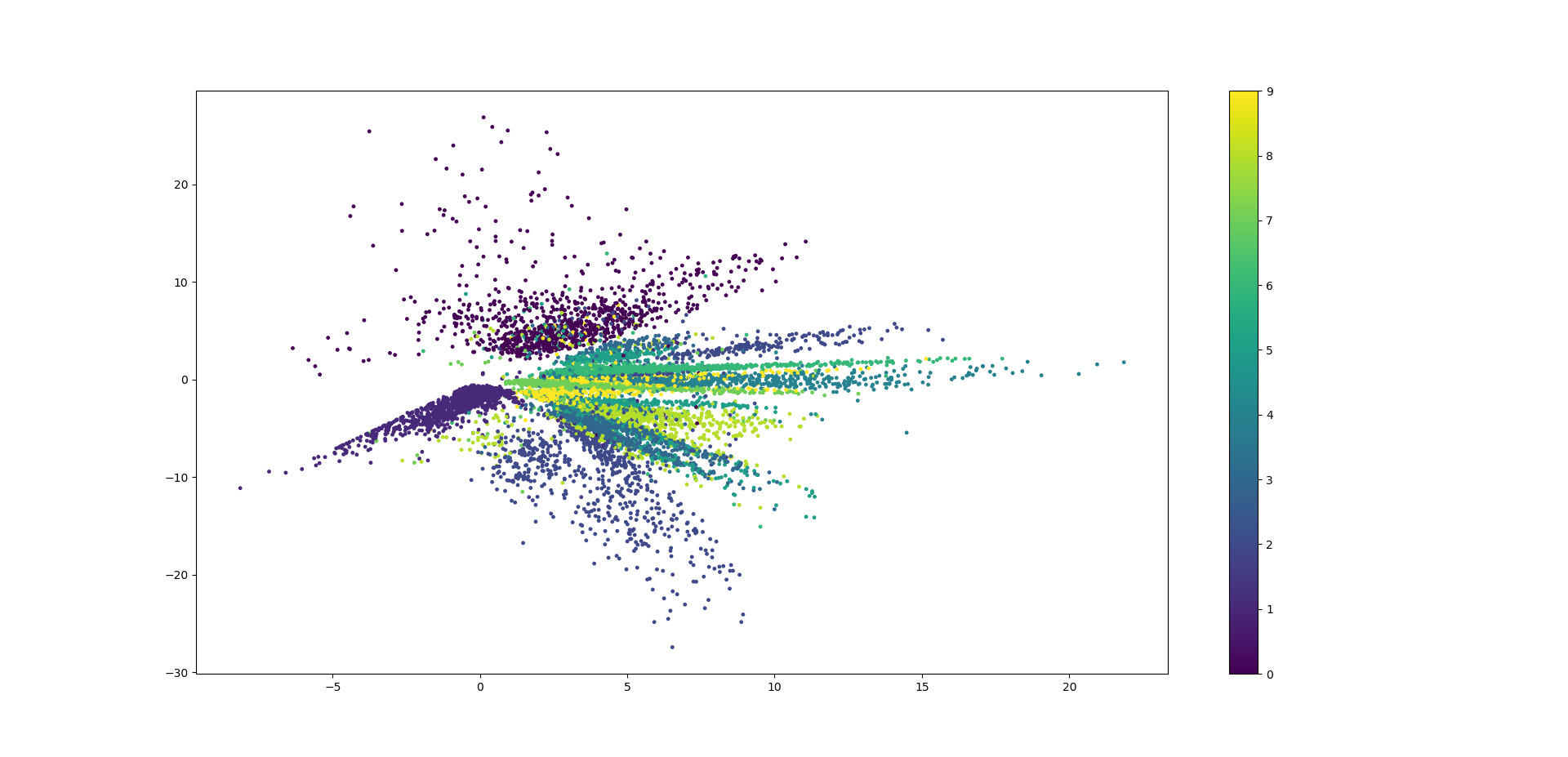# python机器学习库keras——AutoEncoder自编码、特征压缩

python教程全解

keras使用深度网络实现自编码，也就是说对每个样本的n维特征，使用k为特征来表示，实现编码压缩的功能。也实现了特征选择的功能。比如手写体包含754个像素，也就包含754个特征，如果想用两个特征表示。在二维矩阵中就能识别手写体数字该怎么做呢。

import numpy as np
import MNIST
np.random.seed(1337)  # for reproducibility

from keras.datasets import mnist
from keras.models import Model  # 泛型模型
from keras.layers import Dense, Input
import matplotlib.pyplot as plt

X_train, Y_train = MNIST.get_training_data_set(60000, True,False)  # 加载训练样本数据集，和one-hot编码后的样本标签数据集。最大60000
X_test, Y_test = MNIST.get_test_data_set(10000, True,False)  # 加载测试特征数据集，和one-hot编码后的测试标签数据集，最大10000
X_train = np.array(X_train).astype(bool)    # 转化为黑白图
Y_train = np.array(Y_train)
X_test = np.array(X_test).astype(bool)   # 转化为黑白图
Y_test = np.array(Y_test)
print('样本数据集的维度：', X_train.shape,Y_train.shape)   # (600, 784)  (600, 10)
print('测试数据集的维度：', X_test.shape,Y_test.shape)   # (100, 784) (100, 10)

# 压缩特征维度至2维
encoding_dim = 2

# this is our input placeholder
input_img = Input(shape=(784,))

# 编码层
encoded = Dense(128, activation='relu')(input_img)
encoded = Dense(64, activation='relu')(encoded)
encoded = Dense(10, activation='relu')(encoded)
encoder_output = Dense(encoding_dim)(encoded)

# 解码层
decoded = Dense(10, activation='relu')(encoder_output)
decoded = Dense(64, activation='relu')(decoded)
decoded = Dense(128, activation='relu')(decoded)
decoded = Dense(784, activation='tanh')(decoded)

# 构建自编码模型
autoencoder = Model(inputs=input_img, outputs=decoded)

# 构建编码模型
encoder = Model(inputs=input_img, outputs=encoder_output)

# compile autoencoder
autoencoder.compile(optimizer='adam', loss='mse')

# 将训练特征即作为输入又作为输出，这样就同时训练的编码和解码
autoencoder.fit(X_train, X_train, epochs=200, batch_size=256, shuffle=True)

# plotting
encoded_imgs = encoder.predict(X_test)
print(encoded_imgs)
plt.scatter(encoded_imgs[:, 0], encoded_imgs[:, 1], c=Y_test, s=6)
plt.colorbar()
plt.show()